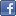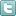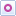EE Times-India > Sensors/MEMS

Sensors/MEMS# Grasping thermistor temperature measurement

Posted: 19 Jun 2015Print Version

Keywords:thermistor  resistance temperature detector  analogue-to-digital converter  ADC  sensors

Temperature is one of the most common parameters monitored by an embedded system. There is a wide array of temperature sensors available to achieve this. The sensor types can range from exotic black body detectors to the simpler more mundane resistance type sensors and everything in between. In this article, I will briefly discuss the negative temperature coefficient (NTC) thermistor—one of the most common temperature sensors used in a wide variety of embedded systems.

Thermistors
A thermistor is a resistance element, usually made from a polymer or semiconductor, where the resistance varies with respect to the temperature. This type of device should not be confused with a resistance temperature detector (RTD). Usually RTDs are much more precise, more expensive, and cover a wider temperature range.

There are two types of thermistors, characterized by how the resistance varies with temperature. If the resistance value lowers with increasing temperature, we call this device a Negative Temperature Coefficient (NTC) thermistor. If the resistance rises with increasing temperature, this device is known as a Positive Temperature Coefficient (PTC) thermistor. In general, PTC devices are used as protection devices and NTC devices are used as thermal sensors. A very common application of NTC thermistors is in sensing and controlling the PN junction of a wide-band laser diode.

Another characteristic of the thermistor is the cost. A typical thermistor in low volume is usually priced in the \$.05 to \$0.10 per piece range. The low cost and ease of interface usually make these devices highly attractive in embedded applications.

A typical thermistor sensing range is from -50 to +125°C. Most thermistor-based applications are used in the -10 to 70°C, or what we know as the typical commercial ambient range.

The typical accuracy of the thermistor is average to fair. Most thermistors are manufactured to yield a ±5% resistance tolerance. The precision of a thermistor, however, is pretty decent. Typically, we can expect to see precision on the order of ±0.5 to ±1.0%.

The resistance transfer equation for the thermistor is known as the Steinhart-Hart equation. This non-linear equation is shown as Equation 1.Equation 1: Steinhart-Hart Equation for a thermistor.

Figure 1 shows the temperature versus resistance plot for a Panasonic ERTJZET472 NTC thermistor. This graph shows that, on a linear scale, the resistance vs. temperature relationship is very non-linear!Figure 1: Resistance vs. Temperature for a Panasonic NTC thermistor.

 Related Articles Editor's Choice
Comment on "Grasping thermistor temperature meas..."

Top Ranked Articles

WebinarsVisit Asia Webinars to learn about the latest in technology and get practical design tips.

Search EE Times India
Services

Go to topConnect on FacebookFollow us on TwitterFollow us on Orkut
﻿